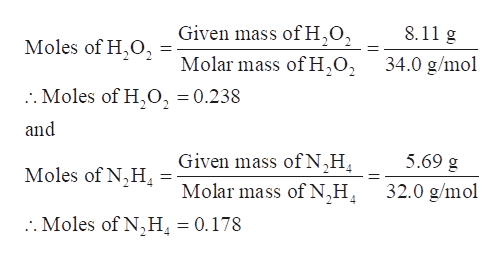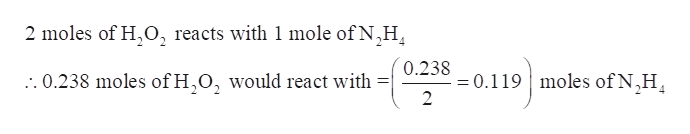# For the chemical reaction shown,2H2O2(l)+N2H4(l)⟶4H2O(g)+N2(g)2H2O2(l)+N2H4(l)⟶4H2O(g)+N2(g)determine how many grams of N2 are produced from the reaction of 8.118.11 g of H2O2 and 5.695.69 g of N2H4.N2 produced:

Question
351 views

For the chemical reaction shown,

2H2O2(l)+N2H4(l)⟶4H2O(g)+N2(g)2H2O2(l)+N2H4(l)⟶4H2O(g)+N2(g)

determine how many grams of N2 are produced from the reaction of 8.118.11 g of H2O2 and 5.695.69 g of N2H4.

N2 produced:

check_circle

Step 1

For the given chemical reaction, the grams of N2 that are produced from the reaction of 8.11 g of H2O2 and 5.69 g of N2H4 are to be determined.

Step 2

Determine the moles of H2O2 and N2H4-

Given mass of H2O2 = 8.11 g

Molar mass of H2O2 = 34.0 g/mol

Given mass of N2H4 = 5.69 g

Molar mass of N2H4 = 32.0 g/molhelp_outlineImage TranscriptioncloseGiven mass of H.O, 8.11 g Moles of H.O2 Molar mass of H,02 34.0 g/mol :. Moles of H,O, = 0.238 and Given mass of N^H 5.69 g Moles of N,H 4 Molar mass of N,H 32.0 g/mol . Moles of N,H, = 0.178 fullscreen
Step 3

Identify the limiting reagent-

As per the balanced equation, 2 moles of H2O2 reacts with 1 mole of N2H4.

Thus, 0.238 moles of H2O2 wo...help_outlineImage Transcriptionclose2 moles of H,O, reacts with 1 mole of N,H 0.238 moles of N,H :. 0.238 moles of H,O, would react with =0.119 2 fullscreen

### Want to see the full answer?

See Solution

#### Want to see this answer and more?

Solutions are written by subject experts who are available 24/7. Questions are typically answered within 1 hour.*

See Solution
*Response times may vary by subject and question.
Tagged in

### General Chemistry